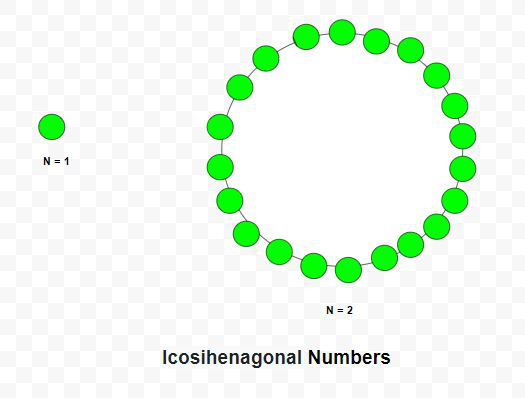Related Articles

# Icosihenagonal Number

• Last Updated : 17 Mar, 2021

Given a number N, the task is to find Nth Icosihenagonal number.

An Icosihenagonal number is class of figurate number. It has 21 – sided polygon called Icosihenagon. The n-th Icosihenagonal number counts the 21 number of dots and all others dots are surrounding with a common sharing corner and make a pattern. The first few Icosihenagonal numbers are 1, 21, 60, 118, 195, 291, 406 …

Examples:

Input: N = 2
Output: 21
Explanation:
The second Icosihenagonal number is 21
Input: N = 6
Output: 291Approach: In mathematics, the Nth Icosihenagonal number is given by the formula:

``

Below is the implementation of the above approach:

## C++

 `// C++ program to find nth``// Icosihenagonal number` `#include ``using` `namespace` `std;` `// Function to find``// Icosihenagonal number``int` `Icosihenagonal_num(``int` `n)``{``    ``// Formula to calculate nth``    ``// Icosihenagonal number``    ``return` `(19 * n * n - 17 * n) / 2;``}` `// Driver Code``int` `main()``{``    ``int` `n = 3;``    ``cout << Icosihenagonal_num(n) << endl;` `    ``n = 10;``    ``cout << Icosihenagonal_num(n) << endl;` `    ``return` `0;``}`

## Java

 `// Java program to find nth``// Icosihenagonal number``class` `GFG{` `// Function to find``// Icosihenagonal number``static` `int` `Icosihenagonal_num(``int` `n)``{``    ``// Formula to calculate nth``    ``// Icosihenagonal number``    ``return` `(``19` `* n * n - ``17` `* n) / ``2``;``}` `// Driver Code``public` `static` `void` `main(String[] args)``{``    ``int` `n = ``3``;``    ``System.out.print(Icosihenagonal_num(n) + ``"\n"``);` `    ``n = ``10``;``    ``System.out.print(Icosihenagonal_num(n) + ``"\n"``);``}``}` `// This code is contributed by Rajput-Ji`

## Python3

 `# Python3 program to find nth``# icosihenagonal number` `# Function to find``# icosihenagonal number``def` `Icosihenagonal_num(n):``    ` `    ``# Formula to calculate nth``    ``# icosihenagonal number``    ``return` `(``19` `*` `n ``*` `n ``-` `17` `*` `n) ``/` `2``    ` `# Driver Code``n ``=` `3``print``(``int``(Icosihenagonal_num(n)))` `n ``=` `10``print``(``int``(Icosihenagonal_num(n)))` `# This code is contributed by divyeshrabadiya07`

## C#

 `// C# program to find nth``// Icosihenagonal number``using` `System;` `class` `GFG{` `// Function to find``// Icosihenagonal number``static` `int` `Icosihenagonal_num(``int` `n)``{``    ``// Formula to calculate nth``    ``// Icosihenagonal number``    ``return` `(19 * n * n - 17 * n) / 2;``}` `// Driver Code``public` `static` `void` `Main()``{``    ``int` `n = 3;``    ``Console.Write(Icosihenagonal_num(n) + ``"\n"``);` `    ``n = 10;``    ``Console.Write(Icosihenagonal_num(n) + ``"\n"``);``}``}` `// This code is contributed by Code_Mech`

## Javascript

 ``
Output:
```60
865```

Attention reader! Don’t stop learning now. Get hold of all the important DSA concepts with the DSA Self Paced Course at a student-friendly price and become industry ready.  To complete your preparation from learning a language to DS Algo and many more,  please refer Complete Interview Preparation Course.

In case you wish to attend live classes with experts, please refer DSA Live Classes for Working Professionals and Competitive Programming Live for Students.

My Personal Notes arrow_drop_up